# SAT Math Multiple Choice Question 969: Answer and Explanation

### Test Information

Question: 969

5.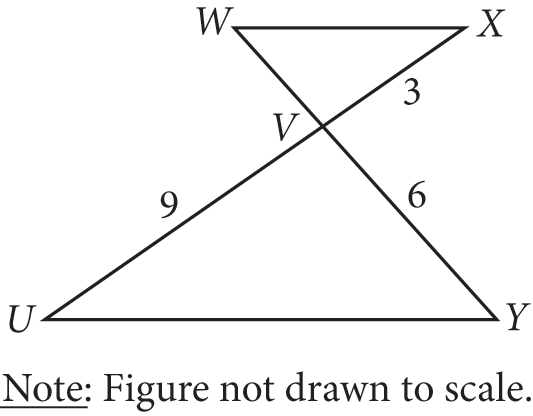In the figure above,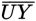and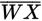are parallel and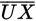intersects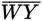at V. What is the length of?

• A. 8
• B. 9
• C. 10
• D. 12

Explanation:

A

Difficulty: Hard

Category: Additional Topics in Math / Geometry

Strategic Advice: When comparing similar (or congruent) triangles that are oriented differently, be sure to mark any corresponding sides and angles with hash marks so that you keep them in the correct order.

Getting to the Answer: Start by filling in as much information as you can on the diagram. ∠WVX is congruent to ∠YVU because they are vertical angles, so mark these angles with a single hash mark. Also note that because UY is parallel to WX, ∠W is congruent to ∠Y, and ∠X is congruent to ∠U (both pairs are alternate interior angles). Mark these pairs with double and triple hash marks. Your diagram might look like the following: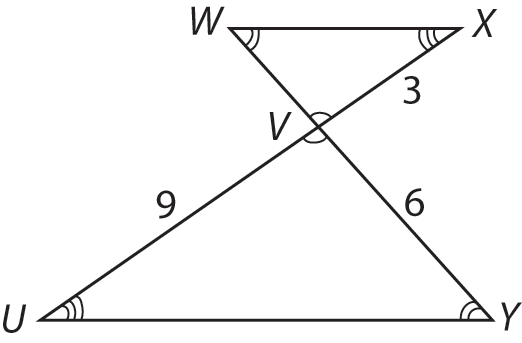Use the hash marks to write a similarity statement-start with the angles marked with one hash mark, then two hash marks, then three: ΔVWX ~ ΔVYU. The order of the letters in the similarity statement makes it clear that VX (first and last letter) corresponds to VU (first and last letter) and is triple the length (based on the figure). Segment VW (first and second letter) is therefore one-third the length of VY (first and second letter), which is 6 ÷ 3 = 2. The question asks for the length of WY, which is 2 + 6 = 8. (A) is the correct answer.